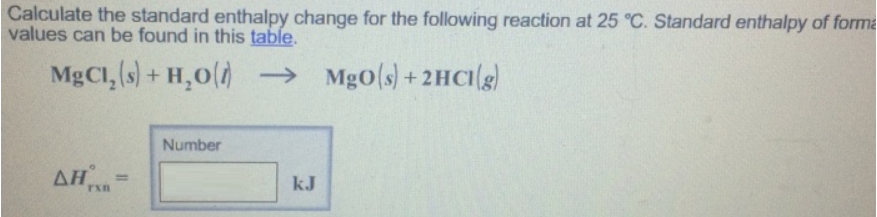# Problem: Calculate the standard enthalpy change for the following reaction at 25°C. Standard enthalpy of forma values can be found in this table. MgCl2(s) + H2O(l) → MgO(s) + 2HCl(g)

###### FREE Expert Solution
97% (110 ratings)###### Problem Details

Calculate the standard enthalpy change for the following reaction at 25°C. Standard enthalpy of forma values can be found in this table.

MgCl2(s) + H2O(l) → MgO(s) + 2HCl(g)